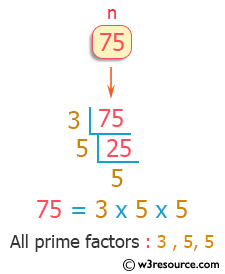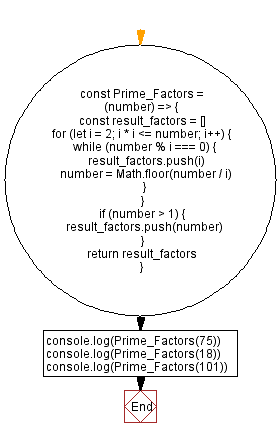# JavaScript: All prime factors of a given number

## JavaScript Math: Exercise-71 with Solution

Write a JavaScript program to print all the prime factors of a given number.

Visualisation:Test Data:
(75) -> [3, 5, 5]
(18) -> [2, 3, 3]
(101) -> 

Sample Solution:

HTML Code:

``````<!DOCTYPE html>
<html>
<meta charset="utf-8">
<title>JavaScript function to all prime factors of a given number</title>
<body>

</body>
</html>
```
```

JavaScript Code:

``````const Prime_Factors = (number) => {
const result_factors = []
for (let i = 2; i * i <= number; i++) {
while (number % i === 0) {
result_factors.push(i)
number = Math.floor(number / i)
}
}
if (number > 1) {
result_factors.push(number)
}
return result_factors
}
console.log(Prime_Factors(75))
console.log(Prime_Factors(18))
console.log(Prime_Factors(101))
```
```

Sample Output:

```[3,5,5]
[2,3,3]

```

Flowchart:Live Demo:

See the Pen javascript-math-exercise-71 by w3resource (@w3resource) on CodePen.

Improve this sample solution and post your code through Disqus

What is the difficulty level of this exercise?

Test your Programming skills with w3resource's quiz.

﻿